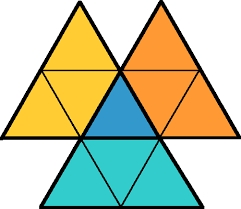# Probability 7991

We have the numbers 4, 6, 9, 13, and 15. What is the probability that these will be the lengths of the sides of the triangle?
(Consider only scalene triangles.)

p =  0.6

### Step-by-step explanation:Did you find an error or inaccuracy? Feel free to write us. Thank you!

Tips for related online calculators
Would you like to compute the count of combinations?